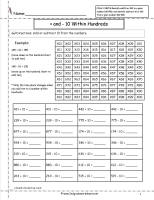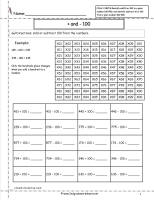﻿ CCSS 2.NBT.8 Worksheets, Adding and Subtracting 10 and 100 From a Given Number

# Common Core State Standard 2.NBT.8

## Number & Operations in Base Ten

### Use place value understanding and properties of operations to add and subtract.

8. Mentally add 10 or 100 to a given number 100–900, and mentally subtract 10 or 100 from a given number 100–900.

###Add and Subtract 10 Worksheet2

Common Core State Standards: 2.NBT.8
Number & Operations in Base Ten
Use place value understanding and properties of operations to add and subtract.

Mentally add 10 or 100 to a given number 100–900, and mentally subtract 10 or 100 from a given number 100–900

Students add and subtract 10 from a number.

###Add and Subtract 100 Worksheet2

Common Core State Standards: 2.NBT.8
Number & Operations in Base Ten
Use place value understanding and properties of operations to add and subtract.

Mentally add 10 or 100 to a given number 100–900, and mentally subtract 10 or 100 from a given number 100–900

Students add and subtract 100 from a number.